# Unifying MATLAB and Simulink: A User Story Part 1

I recently had an interesting discussion with a group of users about modeling, simulation, and how the management of simulation data evolved at their company. What they came up with is a framework where the fundamental unit (which they call a "Part"), is systematically made of a Simulink masked subsystem and a MATLAB class.
Why did they come up with that? To answer this question, I will quote a user from this group: "What really drove MATLAB classes for us was the idea of reuse across system scales. From unit test development up to integration model."
I personally find this framework very convenient to work with, so I thought this would be an interesting story to share on this blog. With their permission, I am sharing the approach I learned from them with all of you.
It's too complex to share in a single post, so I came up with a series of blog posts:
1. Defining model data: From hardcoded values to MATLAB objects (this post)
2. A superclass and template subsystem as foundation of the framework
3. Controlling variants using MATLAB objects
4. Managing logging using MATLAB objects
I will add hyperlinks to this list as posts in this series get published.
I am very curious to hear in the comments below what you think about the approach described in this series of blog posts, and how differently you manage your simulation data and why.
For this first post, I will cover how this group of users evolved their way of defining and specifying data for a Simulink model.

The first time you created a Simulink model, you very likely hardcoded numbers in block dialogs.
You can find such an example model in the first section of the Basic Modeling Workflow: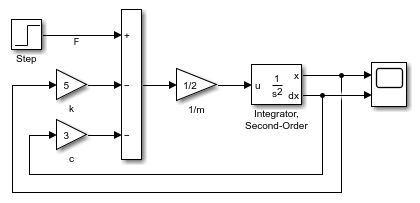As you can imagine, this is not a scalable solution. What if I want to simulate a model with a different set of parameters?

## MATLAB and Simulink 101: Scripts and Variables

The first natural step I see toward data management in Simulink is usually to specify variables in block dialogs, create a MATLAB script to define those variables and evaluate this script in the MATLAB base workspace.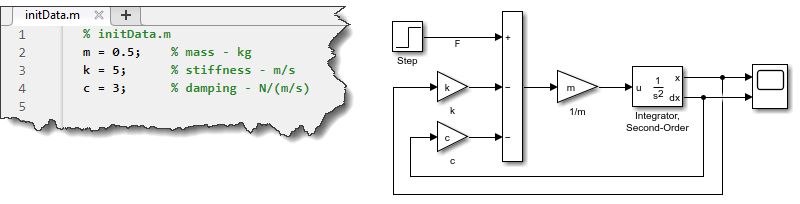This has the advantage of separating the data definition from the model. For example, you could create multiple versions of the MATLAB script to define multiple datasets for the model.
On the other side, this also leads to scalability issues and many potential conflicts, especially in a collaborative environment. What if someone else adds a block with a parameter also named “m” or “k”?

In a similar way, in MATLAB, functions give you a workspace to encapsulate data.
Combining those two concepts, the next step in this evolution is to create a MATLAB function returning a structure containing all the data needed by a masked subsystem.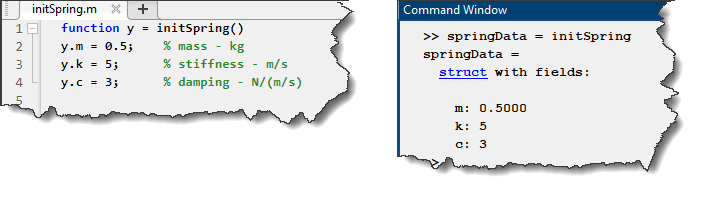In Simulink, the algorithm is masked with a single variable, named "data" in this example, and the structure fields are then used in the individual blocks.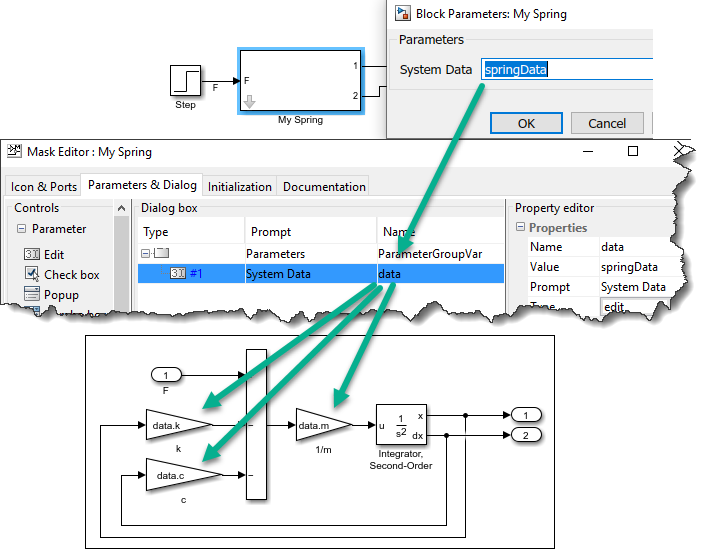That way, if I create a model with multiple instances of this system, I can easily create different data structures.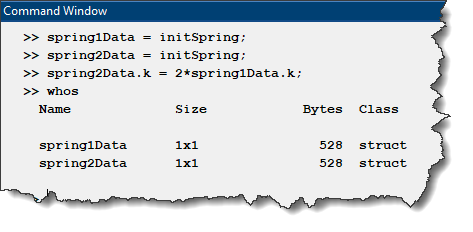and pass them to the different instances of the block.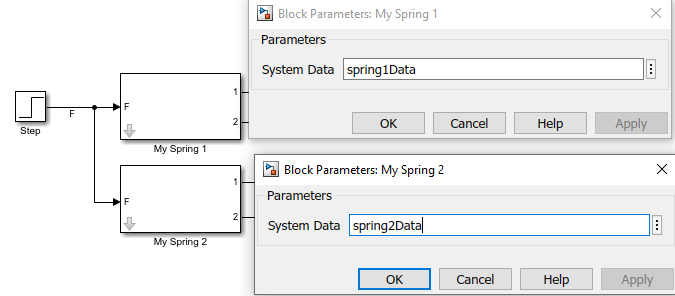### Nested Structures

One great thing with structures is that you can nest them. If you have a complex Simulink model made of multiple components and subcomponents, you can parameterize it in a similar manner, with a MATLAB structure made of sub-structures and sub-sub-structures.
Let's take this model with one subsystem at the top level, ComponentA, is made of two subsystems, My Spring 1 and My Spring 2: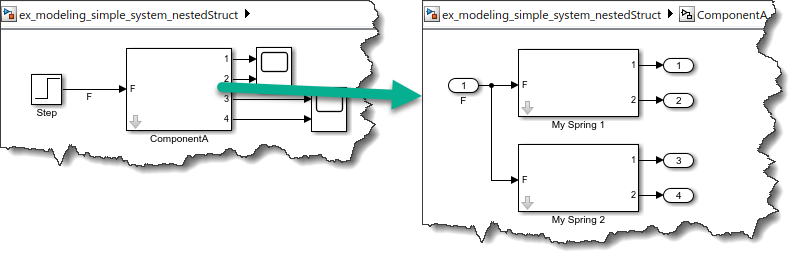In a manner similar to the previous iteration, I can create one function for the top-level component that calls the initialization functions of two subcomponents: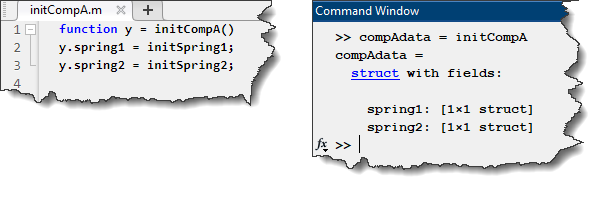and pass that to the masked subsystem containing the two components: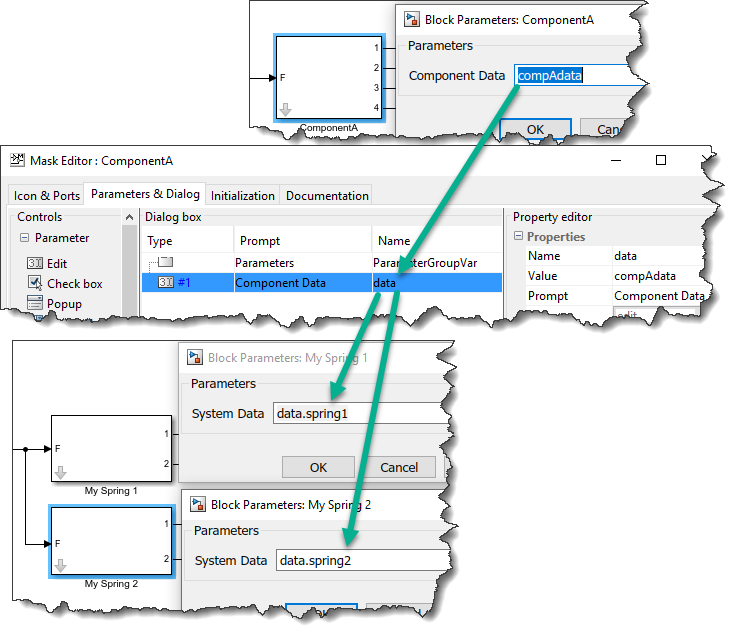At this point there is practically no limit to the width and depth such component hierarchy can have.
At the end of the day, you have a single variable in MATLAB containing a structure similar to the structure of your model.

## MATLAB and Simulink 501: Classes and Objects

The next step in this evolution is transitioning from structures to MATLAB classes. In its simplest form, storing data in the properties of a MATLAB class is very similar to storing it in the fields of a structure.
If we transition our mass-spring-damper example from a structure to a MATLAB class, here is how it would look like: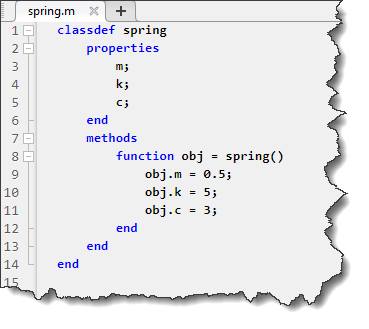Once the class file is created, you can instantiate it and pass the object to the mask parameter just like with a structure.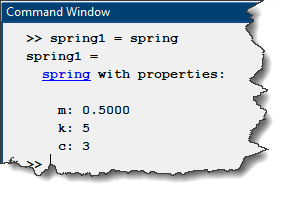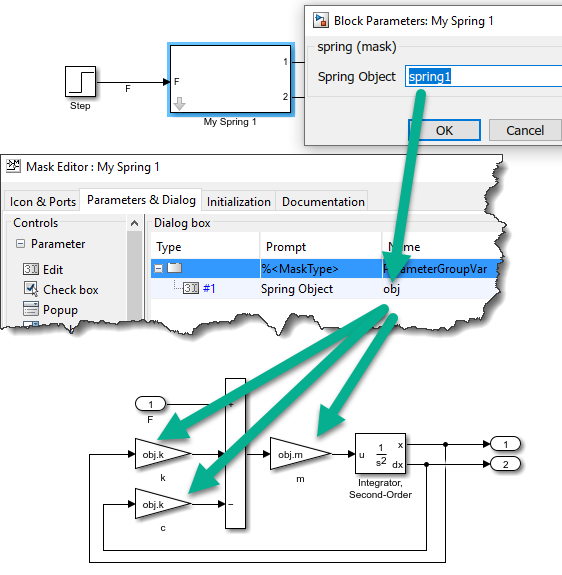In a same way as described above for structures, MATLAB objects can be nested. In that case, an object becomes the property of a parent object. As we did for the structure use case, let's create a component made of two instances of the spring as subcomponents, but with different parameter values: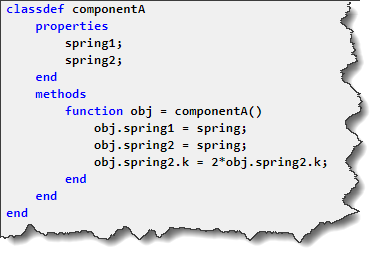Those can then be passed as mask parameters just like structures.
I will not go in details in this post, but MATLAB classes offer multiple advantages when compared to structures. In addition to declaring a formal list of properties, MATLAB classes allow you to define things like custom display and parameter validation methods. For example, you could validate that a parameter is of a specific data type or is within a certain range of values. If you want to read more on this topic I encourage you to see this blog post by Tim Johns on the Developer zone blog: You’ve Got Mail